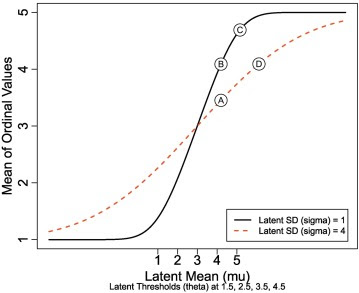## Wednesday, September 19, 2018

### Which movie (treatment, group) is better? Opposite conclusions from different models.

Which movie is better? One way to answer is by considering the star ratings given to those movies. Just treat those 1-to-5 star ratings as numbers, throw them into a t test, and out pops your answer. Right? Not necessarily...

The analogous structure arises in many situations. Suppose, for example, we ask which group is happier, a group of poor people or a group of rich people? One way to answer is by considering subjective happiness ratings from an ordinal scale: 1 = very unhappy, 2 = mildly unhappy, 3 = neither unhappy nor happy, 4 = mildly happy, 5 = very happy. Just treat those 1-to-5 ratings as numbers, throw them into a t test, and out pops your answer. Right? Not necessarily...

Or, consider ratings of symptom intensity in different treatment groups. How bad is your headache? How depressed do you feel? Just treat the ratings as numbers and throw them into a t test, and out pops your answer. Right? Not necessarily...

Treating ordinal values as if they were numeric can lead to misinterpretations. Ordinal values do not indicate equal distances between their levels, nor equal coverage of each level. The conventional t test (and ANOVA and least-squares regression, etc.) assumes the data are metric values normally distributed around the model's predicted values. But obviously ordinal data are not normally distributed metric values.

A much better model of ordinal data is the ordered-probit model, which assumes a continuous latent dimension that is mapped to ordinal levels by slicing the latent dimension at thresholds. (The ordered-probit model is not the only good model of ordinal data, of course, but it's nicely analogous to the t test etc. because it assumes normally distributed noise on the latent dimension.)

The t test and the ordered probit model can produce opposite conclusions about the means of the groups. Here's an example involving star ratings from two movies:

The figure above shows data from two movies, labelled as Cases 5 and 6 in the first two columns. The pink histograms show the frequency distributions of the star ratings; they are the same in the upper and lower rows. The upper row shows the results from the ordered-probit model. The lower row shows the results from the metric model, that is, the t test. In particular, the right column shows the posterior difference of mu's for the two movies The differences are strongly in opposite directions for the two analyses. Each posterior distribution is marked with a dotted line at a difference of zero, and the line is annotated with the percentage of the distribution below zero and above zero. Notice the ordered-probit model fits the data much better than the metric model, as shown by the posterior predictions superimposed on the data: blue dots for the ordered probit model, and blue normal distributions for the metric model. (This is Figure 8 of the article linked below.)

Published article:
https://www.sciencedirect.com/science/article/pii/S0022103117307746

Preprint manuscript: https://osf.io/9h3et/

R code: https://osf.io/53ce9/files/

## Friday, September 7, 2018

### Analyzing ordinal data with metric models: What could possibly go wrong? (just published)

Just published:

Analyzing ordinal data with metric models: What could possibly go wrong?
We surveyed all articles in the Journal of Personality and Social Psychology(JPSP), Psychological Science (PS), and the Journal of Experimental Psychology: General (JEP:G) that mentioned the term “Likert,” and found that 100% of the articles that analyzed ordinal data did so using a metric model. We present novel evidence that analyzing ordinal data as if they were metric can systematically lead to errors. We demonstrate false alarms (i.e., detecting an effect where none exists, Type I errors) and failures to detect effects (i.e., loss of power, Type II errors). We demonstrate systematic inversions of effects, for which treating ordinal data as metric indicates the opposite ordering of means than the true ordering of means. We show the same problems — false alarms, misses, and inversions — for interactions in factorial designs and for trend analyses in regression. We demonstrate that averaging across multiple ordinal measurements does not solve or even ameliorate these problems. A central contribution is a graphical explanation of how and when the misrepresentations occur. Moreover, we point out that there is no sure-fire way to detect these problems by treating the ordinal values as metric, and instead we advocate use of ordered-probit models (or similar) because they will better describe the data. Finally, although frequentist approaches to some ordered-probit models are available, we use Bayesian methods because of their flexibility in specifying models and their richness and accuracy in providing parameter estimates. An R script is provided for running an analysis that compares ordered-probit and metric models.Fig. 4. Ordinal mean as a function of latent mean (mu) and SD (sigma). Groups marked Ⓐ and Ⓑ illustrate a false alarm (Type I error) for which the underlying means are exactly equal but the ordinal means are very different. Groups marked Ⓑ and Ⓓ illustrate a miss (Type II error) for which the underlying means are quite different but the ordinal means are exactly equal. Groups marked Ⓒ and Ⓓ illustrate an inversion for which the underlying means have μD > μC but the ordinal means incorrectly have μC > μD.

Published article:
https://www.sciencedirect.com/science/article/pii/S0022103117307746

Preprint manuscript: https://osf.io/9h3et/

R code: https://osf.io/53ce9/files/